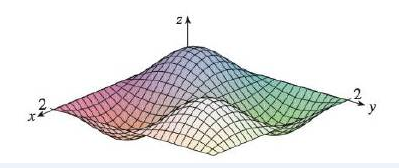Chapter 14, Problem 7RE

Chapter
Section
Textbook Problem

Make a rough sketch of a contour map for the function whose graph is shown.To determine

To sketch: The contour map of the function whose graph is given.

Explanation

Consider the given graph. it has two ups and two dowsn. So the contour map will have 4 partitions. Also, look at the domain of the graph.

Thus, the contour map of the function is shown below in Figure 1.

Still sussing out bartleby?

Check out a sample textbook solution.

See a sample solution

The Solution to Your Study Problems

Bartleby provides explanations to thousands of textbook problems written by our experts, many with advanced degrees!

Get Started

Find more solutions based on key concepts